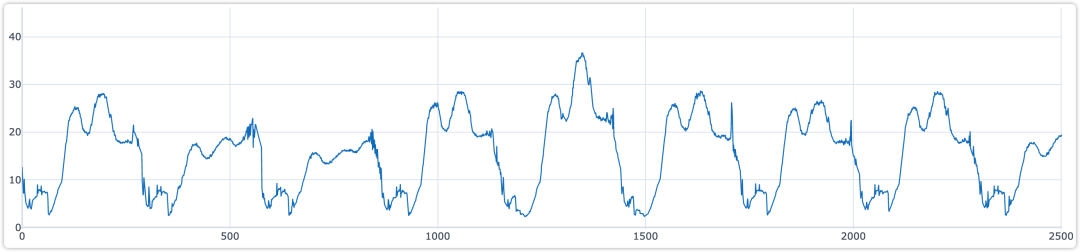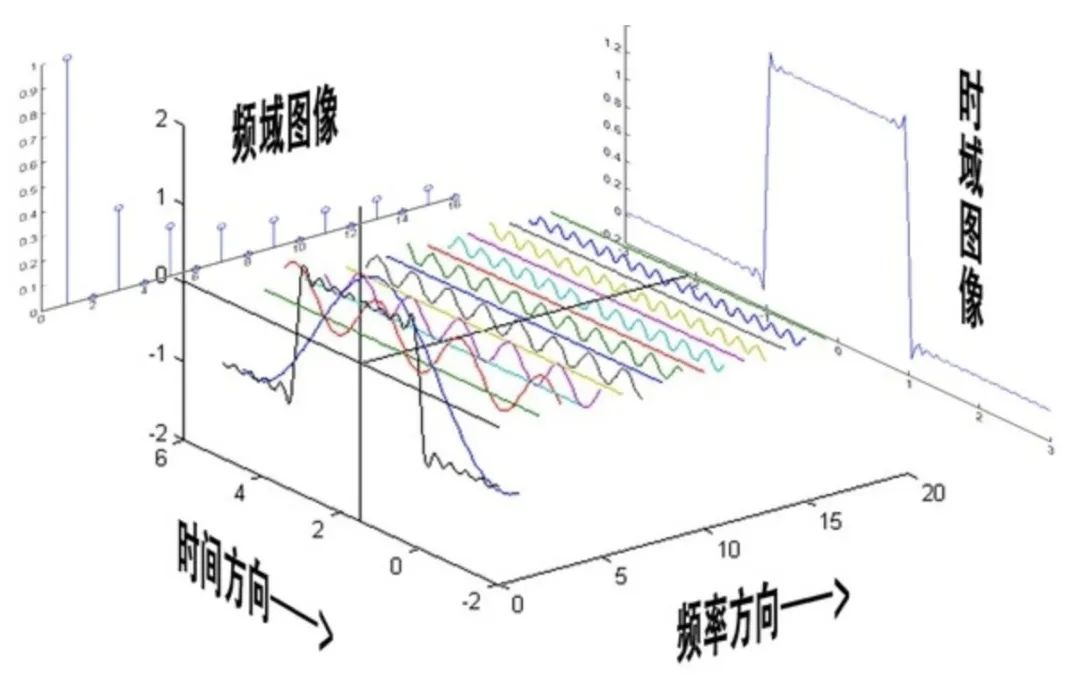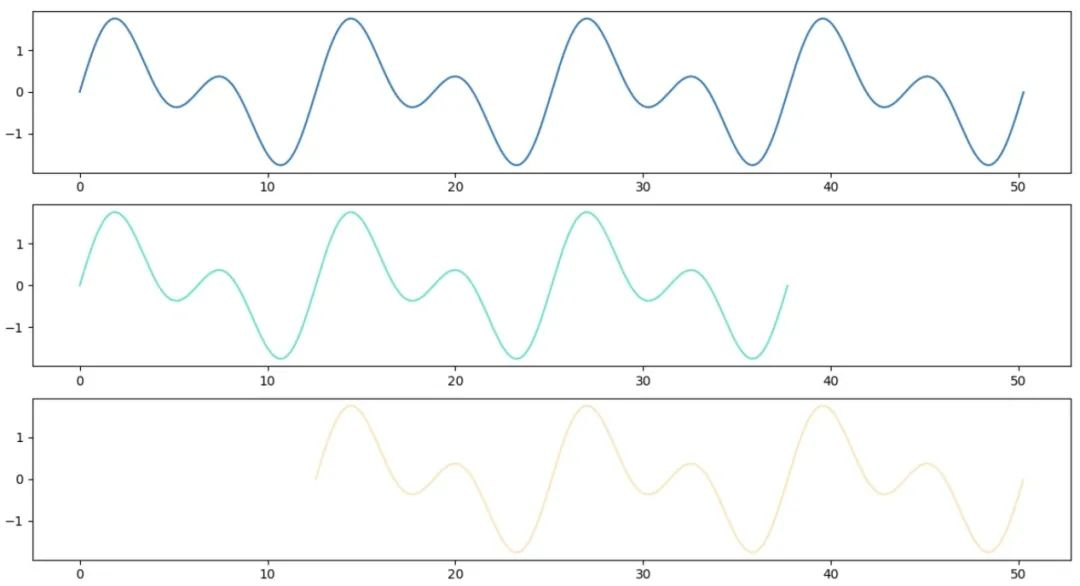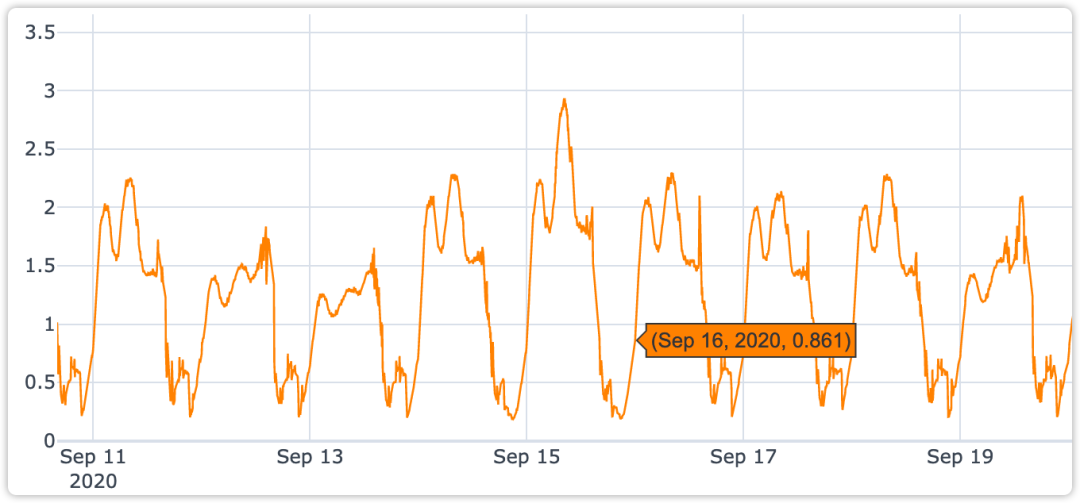【时间序列】周期性检测算法及其 Python 实践

背景• 「符号性周期」，例如序列 fbcnfkgbfopsf 周期为 4；

• 「部分周期性」，例如序列 ansdcdmncdcacdascdmccd 周期为 4；

• 「分段周期性」，例如上面给定的时间序列即为分段周期性；

傅里叶变换自相关系数实例说明from scipy.fftpack import fft, fftfreq

fft_series = fft(data["value"].values)
power = np.abs(fft_series)
sample_freq = fftfreq(fft_series.size)

top_k_seasons = 3
# top K=3 index
top_k_idxs = np.argpartition(powers, -top_k_seasons)[-top_k_seasons:]
top_k_power = powers[top_k_idxs]
fft_periods = (1 / freqs[top_k_idxs]).astype(int)

print(f"top_k_power: {top_k_power}")
print(f"fft_periods: {fft_periods}")

from statsmodels.tsa.stattools import acf

# Expected time period
for lag in fft_periods:
# lag = fft_periods[np.abs(fft_periods - time_lag).argmin()]
acf_score = acf(data["value"].values, nlags=lag)[-1]
print(f"lag: {lag} fft acf: {acf_score}")

expected_lags = np.array([timedelta(hours=12)/timedelta(minutes=5), timedelta(days=1)/timedelta(minutes=5), timedelta(days=7)/timedelta(minutes=5)]).astype(int)
for lag in expected_lags:
acf_score = acf(data["value"].values, nlags=lag, fft=False)[-1]
print(f"lag: {lag} expected acf: {acf_score}")

lag: 72 fft acf: 0.07405431832776994
lag: 278 fft acf: 0.7834457453491087
lag: 292 fft acf: 0.8259822269757922
lag: 144 expected acf: -0.5942986094704665
lag: 288 expected acf: 0.8410792774898174
lag: 2016 expected acf: 0.5936030431473589

【AI蜗牛车出品】手把手AI项目、时空序列、时间序列、白话机器学习、pytorch修炼

03-232978
02-1211-021607
09-03988
06-30880
08-21372
10-276419
03-061万+
04-04678
03-17
05-093146
01-29235
10-276797
08-171万+
03-302871
©️2022 CSDN 皮肤主题：Age of Ai 设计师：meimeiellie点击重新获取扫码支付1.余额是钱包充值的虚拟货币，按照1:1的比例进行支付金额的抵扣。
2.余额无法直接购买下载，可以购买VIP、C币套餐、付费专栏及课程。余额充值